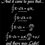# complex angle?

$\cos(i\theta) + i\sin(i\theta)$.

This equation gives a real value $e^{(-\theta)}$. This shows that purely complex number can be equal to purely real number. But for this angle must be complex. Is this possible? Can angle be complex? I think in maths everything is possible. Initially no one knows about $x^2+1=0$. So if angle can be imaginary then we will get our result to be real. So what does it signify?Note by Akash Shukla
4 years, 5 months ago

This discussion board is a place to discuss our Daily Challenges and the math and science related to those challenges. Explanations are more than just a solution — they should explain the steps and thinking strategies that you used to obtain the solution. Comments should further the discussion of math and science.

When posting on Brilliant:

• Use the emojis to react to an explanation, whether you're congratulating a job well done , or just really confused .
• Ask specific questions about the challenge or the steps in somebody's explanation. Well-posed questions can add a lot to the discussion, but posting "I don't understand!" doesn't help anyone.
• Try to contribute something new to the discussion, whether it is an extension, generalization or other idea related to the challenge.

MarkdownAppears as
*italics* or _italics_ italics
**bold** or __bold__ bold
- bulleted- list
• bulleted
• list
1. numbered2. list
1. numbered
2. list
Note: you must add a full line of space before and after lists for them to show up correctly
paragraph 1paragraph 2

paragraph 1

paragraph 2

[example link](https://brilliant.org)example link
> This is a quote
This is a quote
    # I indented these lines
# 4 spaces, and now they show
# up as a code block.

print "hello world"
# I indented these lines
# 4 spaces, and now they show
# up as a code block.

print "hello world"
MathAppears as
Remember to wrap math in $$ ... $$ or $ ... $ to ensure proper formatting.
2 \times 3 $2 \times 3$
2^{34} $2^{34}$
a_{i-1} $a_{i-1}$
\frac{2}{3} $\frac{2}{3}$
\sqrt{2} $\sqrt{2}$
\sum_{i=1}^3 $\sum_{i=1}^3$
\sin \theta $\sin \theta$
\boxed{123} $\boxed{123}$

Sort by:

Well, actually, we have the following definitions $\forall x \in \mathbb{C}$ \begin{aligned} \cos x &= \frac{e^{ix}+e^{-ix}}{2} \\ \sin x &= \frac{e^{ix}-e^{-ix}}{2i}\end{aligned}

The definition of trignometric ratios first started by using angles. Later, it cropped up in other places as well. So, the above analytic definition was accepted. Also, notice that for $x \in \left(0, \dfrac{\pi}{2} \right)$, this definition coincides with the geometric one.

- 4 years, 5 months ago

Thank you, @Deeparaj Bhat . But I still want to know what does it mean?. I mean to say complex number doesn't exist. Yet If we add complex angle to complex number then it results to real 'an existing one'

- 4 years, 5 months ago

The notion of angle is not used in the definition given above. As such, it doesn't make any reference to diagrams.

I don't understand what you mean by 'complex number doesn't exist'.

Also, real numbers are as imaginary as complex numbers. All these number systems are constructed by us. In fact, manier times complex numbers are used in real life situations.

- 4 years, 5 months ago

for existence, I mean to say that we cannot say we have 'i' number of things. Also we cannot get 'i' grams of anything. And in practical life,I think, we always neglect the complex terms. I think we donot make new thing, Only just we observe and do accordingly and it becomes a new thing , then we say that we have constructed this !.

- 4 years, 5 months ago

But you do say 'i' ohms of impedence. For other things, we've not introduced such a notion as it doesn't simplify anything.

No. We do make new things. How do you have decimals in nature? We created it to simplify stuff.

- 4 years, 5 months ago

I think It should be 'we discover new thing'. Our mind doesn't think new things. It just observe nature and discover it. Physics is about observation. And why are you saying about creation. I have question about relation of complex and real numbers in reversible manner.

Decimals in nature. it has also relation with nature. Observation is medium to know about nature. Initially our observation was limited to only different things. So we discovered numbers 1,2,3,. Then we have observed in the particular thing.We thought what is there in the thing? Similarly we thought what is after '1'. For any particular thing, there is always something inside it. In this way decimal may be discovered.

- 4 years, 5 months ago

Well, this isn't physics.

- 4 years, 5 months ago

The last line of question is the main question.

- 4 years, 5 months ago

Like I've already said before, in the modern definition, angles aren't used. It has no physical significance. Also, $\sin$ is imaginary for imaginary values and $\cos$ is real for imaginary values.

- 4 years, 5 months ago

How can it has no significance? Everything has its own significance. Ok, you have explained it.

- 4 years, 5 months ago

If you say so.

- 4 years, 5 months ago